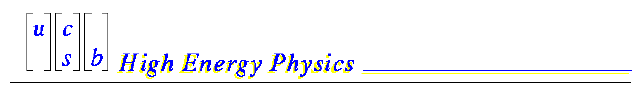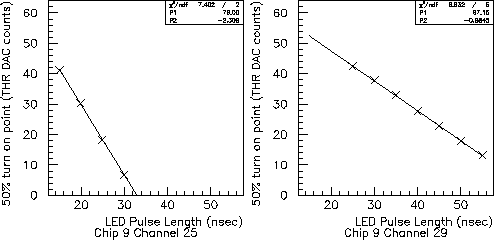# Study of SVT gains

## Introduction

This study uses external charge injection from an infrared LED to address the following questions:
• Possible anomalies in the gain of chips exhibiting "Chip 9 / LG" problems under internal charge injection.

• Possible differences in the charge collection efficiency on the phi side of the inner SVT layers with and without a floating strip.

• Possible differences in charge collection efficiency between the phi (n) and z (p) sides of the inner SVT layers (this is an issue raised by puzzling results of the 1997 test beam analysis).

## Preliminaries

All data here were taken using the layer 1 DFA D01B-6 bonded to HDI H1-18. The z (p) side of this DFA was fully bonded to AToM Rad Hard Version 2 chips. Channels for chips 0, 1, and 2 on the phi (n) side were bonded leaving a floating strip. For the remaining chips, channels 0-63 were fully bonded, channels 64-127 were bonded leaving a floating strip.

All measurements were performed at 100 nsec shaping time, using a 40 MHZ clock and a 3 microsecond trigger jitter window.

The LED light was shined on chip 3 (phi side) in both the bonded and 1/2 bonded regions, and on chip 9 and 10 (z side). Chip 9 is a chip which on this HDI exhibits a "Chip 9 / LG" problem; chip 10 is a good chip. A layer 1 DFA is made up of two separate wafers. In all measurements presented here light was shined always on the first wafer. The bias voltage was 37 V, and the isolation voltage for this wafer is 24 V.

The LED was a HP-HFBR-164T, with a wavelength of 820 nm. The amount of light emitted by the LED was controlled by adjusting the width of the driving pulse between 15 and 55 nsec. The risetime of the LED is of order 5 nsec. The pulse was generated by a front-panel controlled 50 MHZ HP-8116A pulser, allowing for good reproducibility of the pulse width. Its amplitude was 2V, with a 50 Ohm series resistor. Light from the LED was attenuated by an OD=1 Neutral Density Filter (factor of 10 attenuation). Light also had to pass through a 1/8" lucite protecting cover, which we did not bother to remove for these tests (!), and which acted as a convenient mechanical support for the filter. Light was always shined on the phi side of the detector. The size of the pulse was of order 600 micron, FWHM.

## Methodology

The key ingredient to this kind of measurement is good analog resolution. We decided not to use the TOT information because we do not feel that we understand it well enough yet. Therefore, charge collection measurements were performed using threshold scans, much as in internal charge injection. This required careful tuning of the light intensity in order to remain within the limited dynamic range of the THR DAC.

As it has been recently pointed out, at the moment our ability to measure offsets is highly questionable. Therefore, we devised a measurement strategy that is independent of chip offsets. The trick is to be able to control the relative amount of charge deposited by the LED in different runs. This is possible by varying the width of the LED pulse. Indeed, we appear to be operating in a region where the deposited LED charge is linear in the pulse width, see Figure 1.Figure 1: Distribution of 50% threshold turn on point vs the length of the LED pulse for two channels on the p-side. Note that thresholds decrease bottom to top, i.e. THR DAC = 0 is the highest threshold, THR DAC = 63 is the lowest threshold. Channel 25 is at or near the maximum of the light intensity, channel 29 is further away, hence the difference in slopes.

When operating in the linear region, it can be shown that the slopes of fits such as those in Figure 1 measure the product of channel gain times charge collection efficiency, weighted by the profile of the LED pulse. Summing up all of the slopes for all of the channels is equivalent to summing over the (normalized) LED pulse profile. This sum is therefore a relative measure of the (average) product of gain and charge collection efficiency. We will call this quantity eff*g in the remainder of this document.

Thus our procedure is the following :
• For each position of the LED, take 9 threshold scan runs with LED pulses varying between 15 and 55 nsec in 5 nsec steps.

• For each channel, fit the 50% turn on points (in units of THR DAC counts) as a function of LED pulse length to a straight line (see for example Figure 1).

• Obtain a relative measure of eff*g by summing up the slopes of all channels.

• If so desired, extract the relative charge collection efficiency eff by correcting for the gain g. This procedure will be discussed later.

As an illustration, we show in Figure 2 slope distributions as a function of channel number. Note that the readout pitch is 50 micron on the phi side and 100 micron on the z side. From the gaussian fits, the LED spot on the z side is somewhat wider. This is effect is entirely due to our focussing system (this was checked by rotating the lens holder by 90 degrees).Figure 2:Distribution of slopes as a function of channel number the phi side (chip 3) and the z side (chip 9) obtained from a set of 9 runs.

We believe that the main source of uncertainty in our measurents of eff*g comes from the algorithm chosen to sum up the slopes. The measurements in the wings of the distributions in Figure 2 are suspect because they can be affected by noise. In general, we sum the slopes by fitting to a gaussian and calculating the area under the curve. Because the distributions are not perfectly gaussians, the answer that we obtain is affected by the range of the fit. For some reason the phi side distributions are more affected by this problem, as can also be seen from careful inspection of Figure 2. Typically we fit the gaussian for channels that see at least of order 0.5 or 1 fC of charge. The differences in the estimates of the sum of slopes can be as large as +/- 7% in some cases. When quoting results below, we will take these effects into account. In a couple of cases we repeated our measurements under identical conditions and we reproduced results at the 1% level or better.

## Some Comments on Gains and Noise

The capacitance for phi channels with floating strips is smaller than without floating strip because the interstrip capacitance dominates the total capacitance. Because of this change in capacitance, the noise in the floating strip configuration is smaller. It also turns out that the gain of the AToM chip decreases slightly with capacitance. Using charge injection, we measure that the gain with a floating strip is approximately 5% higher than without. In measuring the relative charge collection efficiencies in the two phi configuration it is necessary to correct for this effect.

As an aside, the measured reduction in noise in the floating strip configuration was reported to be approximately 15%. This measurements neglected to properly account for the change in gain. When this is done properly, the reduction in noise becomes 20%. It does not happen very often that the small factors of a few percent add up in the right direction.... but we'll take them...

## Comparison of Floating and Non-Floating Configurations on the Phi Side

This measurement was performed on channels readout by the same chip. Therefore all systematics due to chip-to-chip process variations cancel in the comparison. The largest systematic uncertainty is due to the uncertainty in calculating the sum of slopes. Using different methods we find the following range of values:

eff*g (float) = (1.07 - 1.20) eff*g (no-float)

eff (float) = (1.00 - 1.14) eff (no-float)

where the relative eff was obtained by correcting the measured slopes, channel by channel, by the relative gains measured in internal charge injection. Values for the relative eff above 1.0 are unphysical. We conclude that our measurements are consistent with 100% charge collection efficiency on the floating strips. It is not clear what kind of lower limit we can place on eff without doing a lot more work. At this stage we guess-estimate that eff is greater than something like 0.9.

## Chip 9 / LG study

As mentioned above, chip 9 on HDI H1-18 shows symptoms of the chip 9 / LG problem, whereas chip 10 does not. The systematic uncertainty in the sum of slopes is now small, because the LED spot on the two chips has the identical size and shape. We find the following range of values:

eff*g (Chip 10) = (0.94 - 0.98) eff*g (Chip 9)

This is well within the expected range of chip-to-chip gain variations. If anything, in this case the Chip 9 / LG chip shows a slightly higher gain than a neighboring good chip. We conclude that we do not see any anomaly in the gain of a chip suffering from the Chip 9 / LG problem.

## Comparison of Phi vs Z Charge Collection Efficiency

Here the systematic uncertainty from summing the slopes is worst because the LED spot is not circular. For example we find a systematic difference in the phi vs z ratio of eff*g of 6% when the LED holder is rotated by 90 degrees. The results shown below remove this effect by averaging the two sets of measurements, but an uncertainty due to different summing algorithms remains:

eff*g (phi) = (0.86 - 1.00) eff*g (z)

The measurements are taken on the fully bonded region of chip 3 (phi side) and on chip 10 (z side). Note that on average we expect the gain on the z side to be a little larger due to the lower capacitance. However, the expected chip-to-chip gain variations are probably just as large.

Finally, we can attempt to factor out the gain in order to just compare eff using the charge injection data. However, given the expected chip-to-chip variations in the charge injection capacitor, it is not clear whether this is a useful excercise or not. In any case, we find:

eff (phi) = (0.95 - 1.15) eff (z)

We do not see any evidence of a reduced charge collection efficiency on the z side of a magnitude large enough to explain the 1997 testbeam results.

 Claudio Campagnari Page Last Updated: Aug 12, 1998 Bryan Dahmes Stephen Levy Owen Long